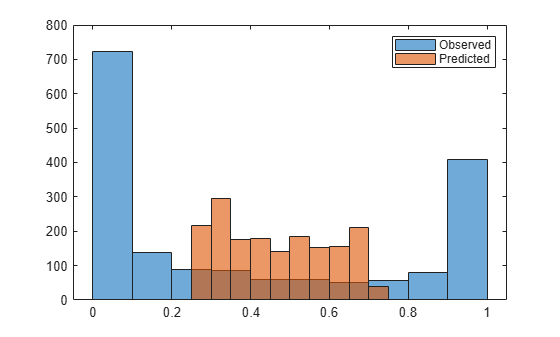# Beta

Create `Beta` model object for exposure at default

Since R2022b

## Description

Create and analyze a `Beta` model object to calculate the exposure at default (EAD) using this workflow:

1. Use `fitEADModel` to create a `Beta` model object.

2. Use `predict` to predict the EAD.

3. Use `modelDiscrimination` to return AUROC and ROC data. You can plot the results using `modelDiscriminationPlot`.

4. Use `modelCalibration` to return the R-squared, RMSE, correlation, and sample mean error of predicted and observed EAD data. You can plot the results using `modelCalibrationPlot`.

## Creation

### Syntax

``BetaEADModel = fitEADModel(data,ModelType)``
``BetaEADModel = fitEADModel(___,Name=Value)``

### Description

example

````BetaEADModel = fitEADModel(data,ModelType)` creates a `Beta` EAD model object.```

example

````BetaEADModel = fitEADModel(___,Name=Value)` specifies options using one or more name-value arguments in addition to the input arguments in the previous syntax. The optional name-value arguments set the model object properties. For example, ```BetaEADModel = fitEADModel(EADData,ModelType,PredictorVars={'UtilizationRate','Age','Marriage'},ConversionMeasure="lcf",DrawnVar='Drawn',LimitVar='Limit',ResponseVar='EAD')``` creates an `BetaEADModel` object using a `Beta` model type. ```

### Input Arguments

expand all

Data for exposure at default, specified as a table.

Data Types: `table`

Model type, specified as a string with the value of `"Beta"` or a character vector with the value of `'Beta'`.

Data Types: `char` | `string`

Name-Value Arguments

Specify optional pairs of arguments as `Name1=Value1,...,NameN=ValueN`, where `Name` is the argument name and `Value` is the corresponding value. Name-value arguments must appear after other arguments, but the order of the pairs does not matter.

Example: ```BetaEADModel = fitEADModel(EADData,ModelType,PredictorVars={'UtilizationRate','Age','Marriage'},ConversionMeasure="lcf",LimitVar='Limit',ResponseVar='EAD',BoundaryTolerance=1e5)```

User-defined model ID, specified as `ModelID` and a string or character vector. The software uses the `ModelID` text to format outputs and is expected to be short.

Data Types: `string` | `char`

User-defined description for model, specified as `Description` and a string or character vector.

Data Types: `string` | `char`

Predictor variables, specified as `PredictorVars` and a string array or cell array of character vectors. `PredictorVars` indicates which columns in the `data` input contain the predictor information. By default, `PredictorVars` is set to all the columns in the `data` input except for `ResponseVar`.

Data Types: `string` | `cell`

Response variable, specified as `ResponseVar` and a string or character vector. The response variable contains the EAD data and must be a numeric variable. By default, `ResponseVar` is set to the last column.

Data Types: `string` | `char`

Value to perturb EAD response values away from 0 to 1, specified as `BoundaryTolerance` and a positive scalar numeric.

Data Types: `double`

Limit variable, specified as `LimitVar` and a string or character vector. `LimitVar` indicates which column in `data` contains the limit amount. The limit amount value in the `data` must be a positive numeric value. The limit depends on the loan. If the loan is a credit card, the limit is the credit limit. If the loan is a mortgage, the limit is the initial loan amount. In general, `LimitVar` is the maximum amount that can be borrowed.

Note

`LimitVar` is required when `ConversionMeasure` is `'lcf'`. For more information on LCF, see Conversion Measure Options.

Data Types: `string` | `char`

Drawn variable, specified as `DrawnVar` and a string or character vector. `DrawnVar` is the balance on the account at the time of observation, before default, and EAD is the balance at the time of default. `DrawnVar` indicates which column in `data` contains the drawn amount. The drawn variable value in the `data` can be a positive or negative numeric value.

Note

When the `ConversionMeasure` is `'lcf'`, `DrawnVar` is not required. In this case, `DrawnVar` is set to `""`.

Data Types: `string` | `char`

Response transform, specified as `ConversionMeasure` and a character vector or string. Limit conversion factor (LCF) is a fraction of the limit representing the total exposure. The EAD is then defined as the LCF times the limit (`EAD = LCF*Limit`).

Data Types: `string` | `char`

Options for fitting, specified as `SolverOptions` and an `optimoptions` object that is created using `optimoptions` from Optimization Toolbox™. The defaults for the `optimoptions` object are:

• `"Display"``"none"`

• `"Algorithm"``"quasi-newton"`

• `"MaxFunctionEvaluations"``500` ✕ Number of model coefficients

• `"MaxIterations"` — 1000

Note

When using `optimoptions` with a `Beta` model, specify the `SolverName` as `fminunc`.

The number of `Beta` model coefficients is determined at run time, depending on the number of predictors and the number of categories in the categorical predictors.

Data Types: `object`

## Properties

expand all

User-defined model ID, returned as a string.

Data Types: `string`

User-defined description, returned as a string.

Data Types: `string`

Underlying statistical model, returned as a compact linear model object. The compact version of the underlying regression model is an instance of the `risk.internal.credit.BetaModel` class.

Data Types: `object`

Predictor variables, returned as a string array.

Data Types: `string`

Response variable, returned as a string.

Data Types: `string`

Limit variable, returned as a string.

Data Types: `string`

Drawn variable, returned as a string.

Data Types: `string`

Response transform, returned as a string.

Data Types: `string`

Value to perturb LGD response values away from 0 to 1, returned as a positive scalar numeric.

Data Types: `double`

## Object Functions

 `predict` Predict exposure at default `modelDiscrimination` Compute AUROC and ROC data `modelDiscriminationPlot` Plot ROC curve `modelCalibration` Compute R-square, RMSE, correlation, and sample mean error of predicted and observed EADs `modelCalibrationPlot` Scatter plot of predicted and observed EADs

## Examples

collapse all

This example shows how to use `fitEADModel` to create a `Beta` model object for exposure at default (EAD).

```load EADData.mat head(EADData)```
``` UtilizationRate Age Marriage Limit Drawn EAD _______________ ___ ___________ __________ __________ __________ 0.24359 25 not married 44776 10907 44740 0.96946 44 not married 2.1405e+05 2.0751e+05 40678 0 40 married 1.6581e+05 0 1.6567e+05 0.53242 38 not married 1.7375e+05 92506 1593.5 0.2583 30 not married 26258 6782.5 54.175 0.17039 54 married 1.7357e+05 29575 576.69 0.18586 27 not married 19590 3641 998.49 0.85372 42 not married 2.0712e+05 1.7682e+05 1.6454e+05 ```
```rng('default'); NumObs = height(EADData); c = cvpartition(NumObs,'HoldOut',0.4); TrainingInd = training(c); TestInd = test(c);```

Select Model Type

Select a model type for `Beta`.

`ModelType =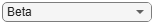"Beta";`

Select Conversion Measure

Select a conversion measure for the EAD response values.

`ConversionMeasure =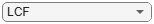"LCF";`

Create `Beta` EAD Model

Use `fitEADModel` to create a `Beta` model object using the `TrainingInd` data.

```BetaEADModel = fitEADModel(EADData(TrainingInd,:),ModelType,PredictorVars={'UtilizationRate','Age','Marriage'}, ... ConversionMeasure=ConversionMeasure,LimitVar="Limit",ResponseVar="EAD",BoundaryTolerance=2e-05); disp(BetaEADModel);```
``` Beta with properties: BoundaryTolerance: 2.0000e-05 ModelID: "Beta" Description: "" UnderlyingModel: [1x1 risk.internal.credit.BetaModel] PredictorVars: ["UtilizationRate" "Age" "Marriage"] ResponseVar: "EAD" LimitVar: "Limit" DrawnVar: "" ConversionMeasure: "lcf" ```

Display the underlying model. The underlying model's response variable is the transformation of the EAD response data. Use the `'LimitVar'` and `'DrawnVar'` name-value arguments to modify the transformation.

`disp(BetaEADModel.UnderlyingModel);`
```Beta regression model: logit(EAD_lcf) ~ 1_mu + UtilizationRate_mu + Age_mu + Marriage_mu log(EAD_lcf) ~ 1_phi + UtilizationRate_phi + Age_phi + Marriage_phi Estimated coefficients: Estimate SE tStat pValue __________ _________ _________ __________ (Intercept)_mu -0.68477 0.1145 -5.9807 2.5234e-09 UtilizationRate_mu 1.7029 0.077717 21.912 0 Age_mu -0.0056329 0.0027489 -2.0492 0.040543 Marriage_not married_mu -0.025614 0.051927 -0.49328 0.62186 (Intercept)_phi -0.46429 0.095342 -4.8697 1.1838e-06 UtilizationRate_phi 0.41621 0.06701 6.2112 6.0942e-10 Age_phi -0.001282 0.0023261 -0.55114 0.58159 Marriage_not married_phi 0.00014873 0.042884 0.0034682 0.99723 Number of observations: 2627 Log-likelihood: -2931.19 ```

EAD prediction operates on the underlying compact statistical model and then transforms the predicted values back to the EAD scale. You can specify the `predict` function with different options for the `'ModelLevel'` name-vale argument.

`predictedEAD = predict(BetaEADModel,EADData(TestInd,:))`
```predictedEAD = 1751×1 105 × 0.1758 0.1029 0.1528 0.0832 0.3261 0.5148 0.0648 0.0531 0.0712 0.3215 ⋮ ```

For model validation, use `modelDiscrimination`, `modelDiscriminationPlot`, `modelCalibration`, and `modelCalibrationPlot`.

Use `modelDiscrimination` and then `modelDiscriminationPlot` to plot the ROC curve.

```ModelLevel =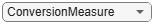"ConversionMeasure"; [DiscMeasure1,DiscData1] = modelDiscrimination(BetaEADModel,EADData(TestInd,:),ModelLevel=ModelLevel); modelDiscriminationPlot(BetaEADModel,EADData(TestInd, :),ModelLevel=ModelLevel,SegmentBy="Marriage");```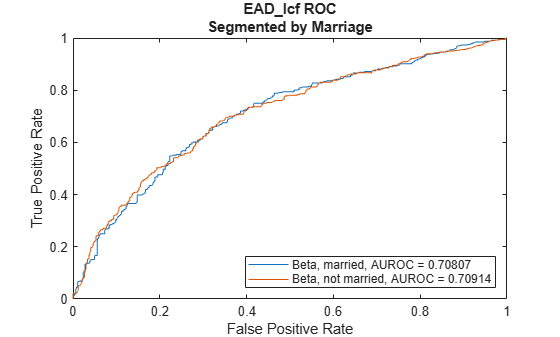Use `modelCalibration` and then `modelCalibrationPlot` to show a scatter plot of the predictions.

```YData =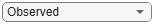"Observed"; [CalMeasure1,CalData1] = modelCalibration(BetaEADModel,EADData(TestInd,:),ModelLevel=ModelLevel); modelCalibrationPlot(BetaEADModel,EADData(TestInd,:),ModelLevel=ModelLevel,YData=YData);```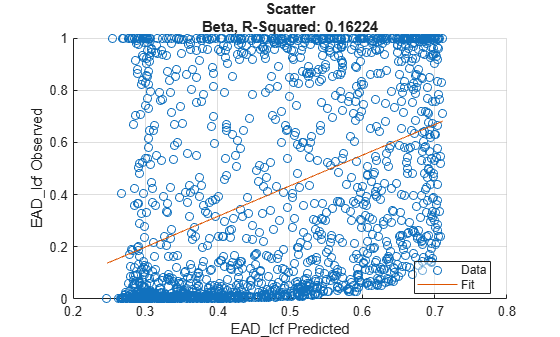Plot a histogram of observed EAD with respect to the predicted EAD.

```figure; histogram(CalData1.Observed); hold on; histogram(CalData1.(('Predicted_' + ModelType))); legend('Observed','Predicted');```